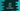# Java program to print a plus star pattern## Java program to print a plus star pattern:

In this post, we will write one program to print the plus pattern with star or any other character in Java. This program will read the size of the pattern as input from the user and print the pattern. Before we start writing the program, let me show you how the algorithm works.

### Algorithm to print a plus star pattern:

Let’s have a look at the below plus pattern:

``````     *
*
*
*
*
***********
*
*
*
*
*``````

This pattern is of height 6. We are using blank space and stars to print the pattern. To understand, how the blank spaces are printed, let me replace all blank spaces with #:

``````#####*
#####*
#####*
#####*
#####*
***********
#####*
#####*
#####*
#####*
#####*``````

To print this pattern, we will use one outer for loop and two inner for loops.

• The outer loop will run from i = 1 to i = 2*n - 1
• In this loop,
• If i is equal to n, run one loop for 2*n - 1 time to print the stars.
• Else, print one loop from j = 1 to j = n. If j is equal to n, print a star. Else, print a blank space.
• Add a new-line at the end of each

### Example 1: Java program to print a plus pattern with star and for loops:

The below program uses the above algorithm to print a plus pattern with star.

``````import java.util.Scanner;

public class Main {

public static void main(String[] args) {
Scanner scanner = new Scanner(System.in);
System.out.println("Enter the size of the pattern : ");
int size = scanner.nextInt();

for (int i = 1; i <= 2 * size - 1; i++) {

if (i == size) {
for (int j = 1; j <= 2 * size - 1; j++) {
System.out.print("*");
}
} else {
for (int j = 1; j <= size; j++) {
if (j == size)
System.out.print("*");
else
System.out.print(" ");
}
}

System.out.println();
}
}
}``````

This program is using the same algorithm explained above. It is using a Scanner object to read the user-input size value.

Sample output:

``````Enter the size of the pattern :
5
*
*
*
*
*********
*
*
*
*

Enter the size of the pattern :
7
*
*
*
*
*
*
*************
*
*
*
*
*
*``````

### Example 2: Java program to print a plus pattern with star and while loops:

The above program can be modified to use while loops. The below program uses while loops to print the pattern:

``````import java.util.Scanner;

public class Main {

public static void main(String[] args) {
Scanner scanner = new Scanner(System.in);
System.out.println("Enter the size of the pattern : ");
int size = scanner.nextInt();
int i = 1, j = 2;
while (i <= 2 * size - 1) {

if (i == size) {
j = 1;
while (j <= 2 * size - 1) {
System.out.print("*");
j++;
}
} else {
j = 1;
while (j <= size) {
if (j == size)
System.out.print("*");
else
System.out.print(" ");
j++;
}
}

System.out.println();
i++;
}
}
}``````

With while loops, we have to initialize the variable before the loop starts and increment its value at the end of the loop. It will print similar results.

### Example 3: Java program to print a plus pattern with any character:

We can take a character as input from the user and use that character to print the pattern.

``````import java.util.Scanner;

public class Main {

public static void main(String[] args) {
Scanner scanner = new Scanner(System.in);
System.out.println("Enter the size of the pattern : ");
int size = scanner.nextInt();

System.out.println("Enter the character to print the pattern: ");
char c = scanner.next().charAt(0);

for (int i = 1; i <= 2 * size - 1; i++) {

if (i == size) {
for (int j = 1; j <= 2 * size - 1; j++) {
System.out.print(c);
}
} else {
for (int j = 1; j <= size; j++) {
if (j == size)
System.out.print(c);
else
System.out.print(" ");
}
}

System.out.println();
}
}
}``````

We added a new character variable c, which is the character used in the pattern. This value is taken as an input from the user. It will use any character to print the pattern:

``````Enter the size of the pattern :
5
Enter the character to print the pattern:
#
#
#
#
#
#########
#
#
#
#``````